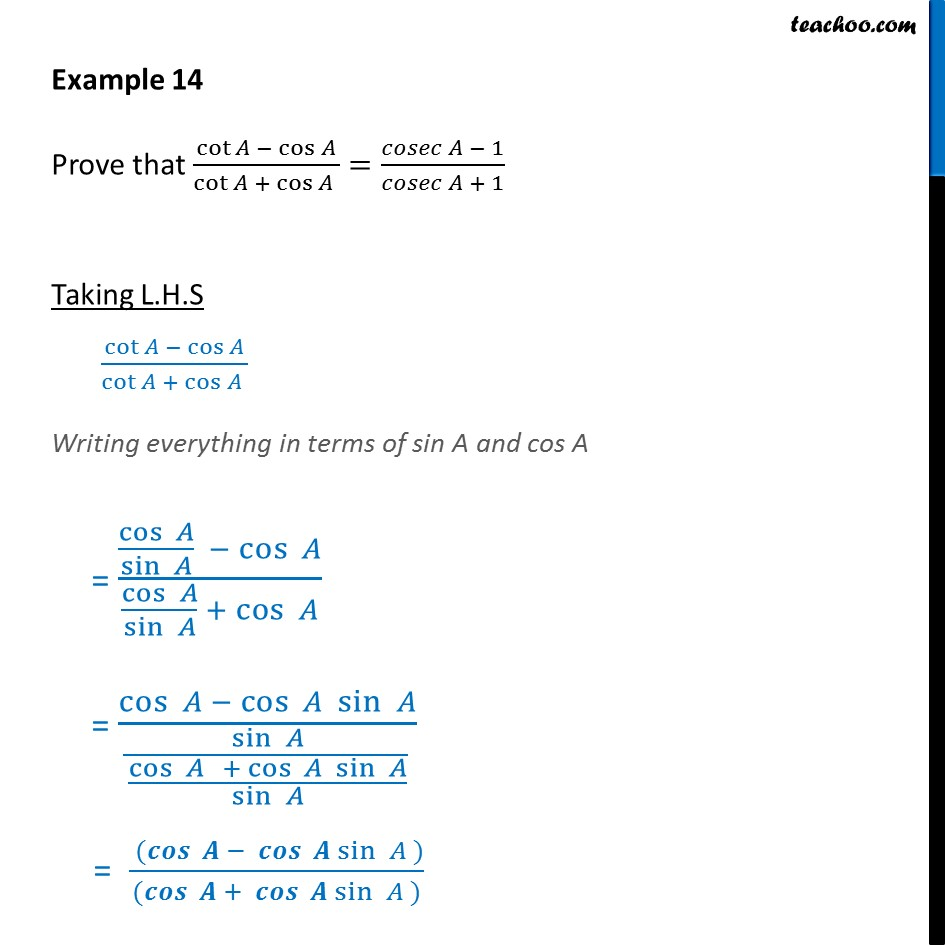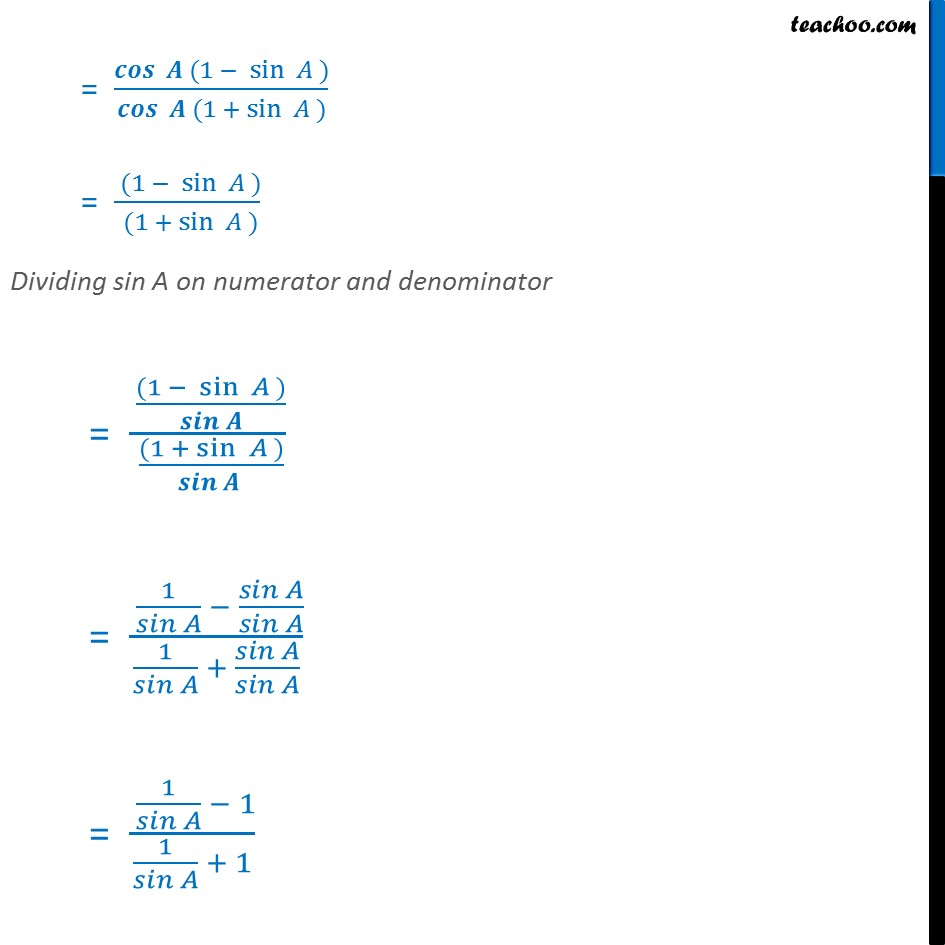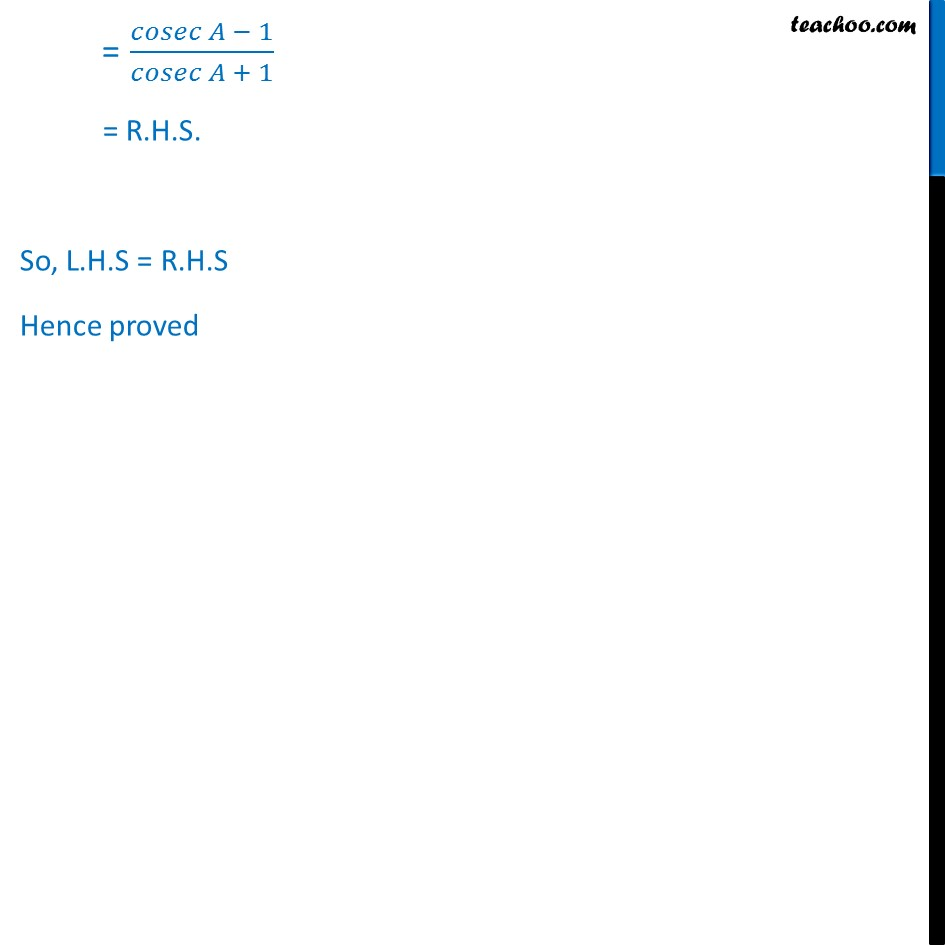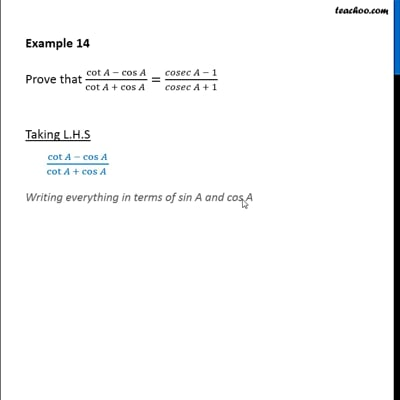Examples

Chapter 8 Class 10 Introduction to Trignometry
Serial order wiseThis video is only available for Teachoo black users

Get live Maths 1-on-1 Classs - Class 6 to 12

### Transcript

Example 14 Prove that cot cos /cot + cos =( 1)/( + 1) Taking L.H.S cot cos /cot + cos Writing everything in terms of sin A and cos A = (cos /sin cos )/(cos /sin + cos ) = cos cos sin /(sin /(cos + cos sin /sin )) = ( ( sin ))/(( + sin )) = ( (1 sin ))/( (1 + sin )) = ( (1 sin ))/( (1 + sin )) Dividing sin A on numerator and denominator = ( ((1 sin ))/( ))/( ((1 + sin ))/( )) = ( 1/( ) ( )/( ))/(1/( ) + ( )/( )) = ( 1/( ) 1)/(1/( ) + 1) = ( 1)/( + 1) = R.H.S. So, L.H.S = R.H.S Hence proved